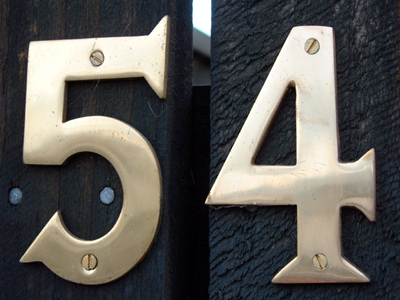54 is the next number of the sequence 18, 27, 36, 45.

# Number Sequences (Year 5)

In KS2 Maths, as you move to Year Five, you'll get really good at spotting number patterns. You'll learn to see if the numbers are going up or down by a certain amount in each step. Odd and even numbers will be like your friends, and you'll even tackle sequences with decimal numbers and fractions!

Finding the next number in a sequence is like solving a puzzle. Some patterns are simple, like adding 10, but others, with decimals or fractions, might seem tricky at first. Don't worry! With a bit of practice, you'll become a pro at spotting patterns in sequences, whether they're made of whole numbers or tricky decimals and fractions.

1.
What will be the tenth number of this sequence? 22, 33, 44, 55, 66
108
110
115
121
The numbers are increasing by 11
2.
What is the next number of the following sequence? 64, 56, 48, 40, 32
35
28
26
24
The numbers are decreasing by 8
3.
What is the next number of the following sequence? 18, 27, 36, 45
50
52
54
56
The numbers are increasing by 9
4.
Can you find the rule of this sequence? 6, 12, 18, 24, 30
The numbers increase in 6s
The numbers increase in 3s
The numbers increase in 5s
The numbers increase in 4s
This sequence is the 6 times table
5.
Can you find the rule of this sequence? 0, 0.1, 0.2, 0.3, 0.4
The numbers increase in 0.1s
The numbers increase in 0.5s
The numbers increase in 1s
The numbers increase in 0.2s
The sequence would continue: 0.5, 0.6, 0.7, 0.8... etc
6.
The sum of three even numbers will be what?
Even
Odd
It will end in 0
It will end in 5
The sum of 2 even numbers will be even. If you add 2 of the 3 numbers and then add another even it is the same as adding 2 even numbers
7.
The difference between two odd numbers is what?
Sometimes odd and sometimes even
Always odd
Always even
It always ends in 0
An odd + an even = an odd so an odd - an odd = an even
8.
The sum of three odd numbers will be what?
Even
Odd
It will end in 0
It will end in 5
The sum of two odd numbers is even but add another odd number and the total will be odd
9.
The difference between an odd number and an even number is what?
Sometimes odd and sometimes even
Always odd
Always even
It always ends in 0
An odd + an odd = an even so an even - an odd = an odd
10.
Can you find the rule of this sequence? 14, 21, 28, 35
The numbers increase in 6s
The numbers increase in 7s
The numbers increase in 5s
The numbers increase in 9s
All of the numbers in this sequence are found in the 7 times table
You can find more about this topic by visiting BBC Bitesize - What is a number sequence?

Author:  Amanda Swift